CoCalc Public Files004 - Handouts / HW10 Solutions / hw10.sagews.html
Authors: Mutiara Sondjaja, Drew Youngren
Views : 64
Compute Environment: Ubuntu 18.04 (Deprecated)
(File too big to render with math typesetting.)
Homework 10 Solutions

# Homework 10 Solutions

 Author Drew Youngren Date 2016-12-06T19:24:09 Project 5ec22442-8b78-4da2-a3f7-b48ea5295fe9 Location 004 - Handouts/HW10 Solutions/hw10.sagews Original file hw10.sagews

# Homework 10 Solutions

(1) How many edges does $K_n$, the complete graph on $n$ vertices, have?

Every size-2 subset of the vertices is an edge, so $K_n$ has $\begin{pmatrix} n \\ 2 \end{pmatrix}$ edges.

(2) How many graphs can be made on the set of vertices $\{1,2,3,\dots,n\}$?

$K_n$ has $\begin{pmatrix} n \\ 2 \end{pmatrix}$ edges, so any subset of them constitutes a unique graph. Thus, there are $2^{\begin{pmatrix} n \\ 2 \end{pmatrix}}$ grraphs.

(3) Let $\sim$ be the relation “is isomorphic to” on the set of all graphs with 4 vertices. Find a representative of each equivalence class. Hint: Organize them by number of edges.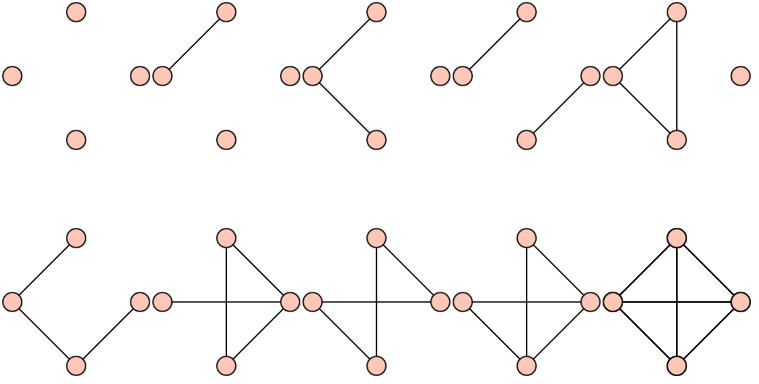(4) Exhibit two graphs that have the same number of vertices and edges but are not isomorphic.

Bonus: Exhibit two graphs with the same number of vertices and edges and the same number of vertices of a given degree, but are not isomorphic.

(5) Plot your answers here. See intro_to_graphs.sagews (start at line 49) for more examples, but the general format is:

Graph([list_of_vertices,list_of_edges])

Modify the example below to fit your needs.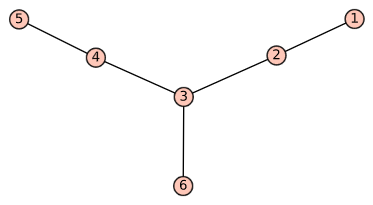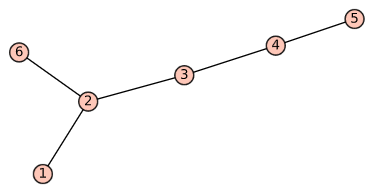False

(6–10) From Steinerman, do exercises §48.6,8,9,10,11 (p. 343).

•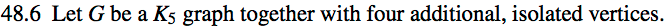•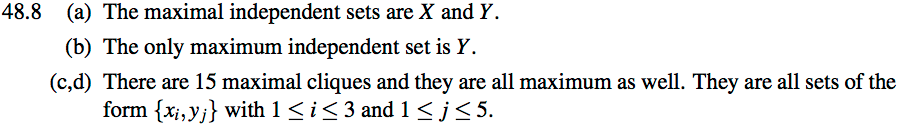•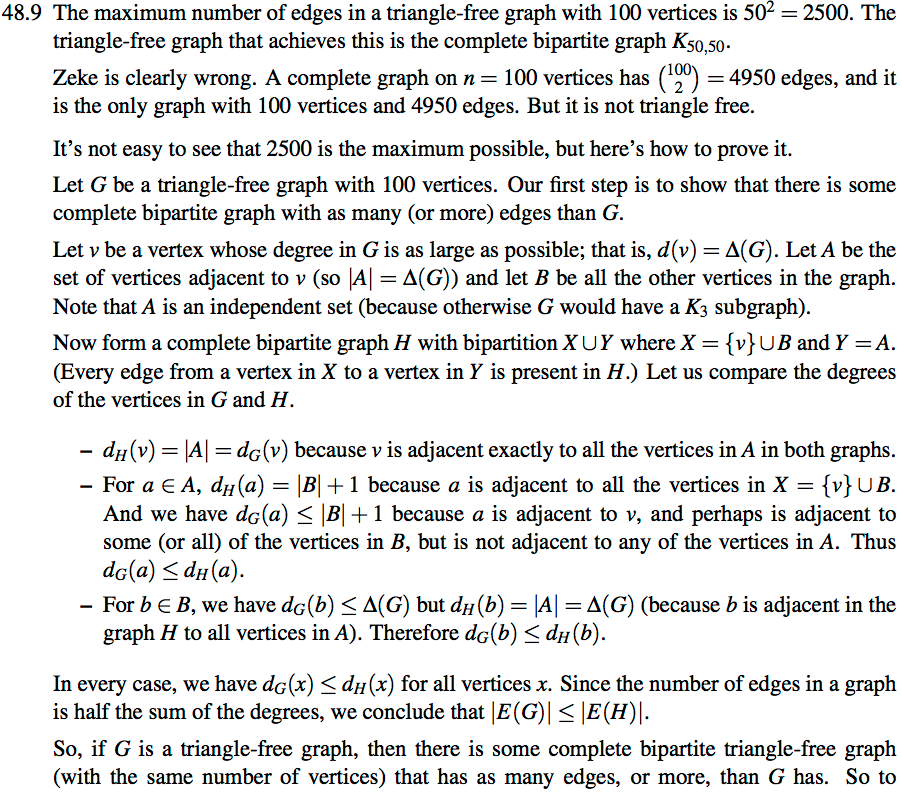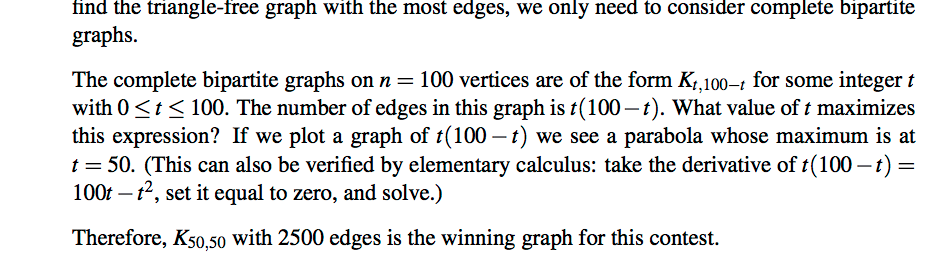•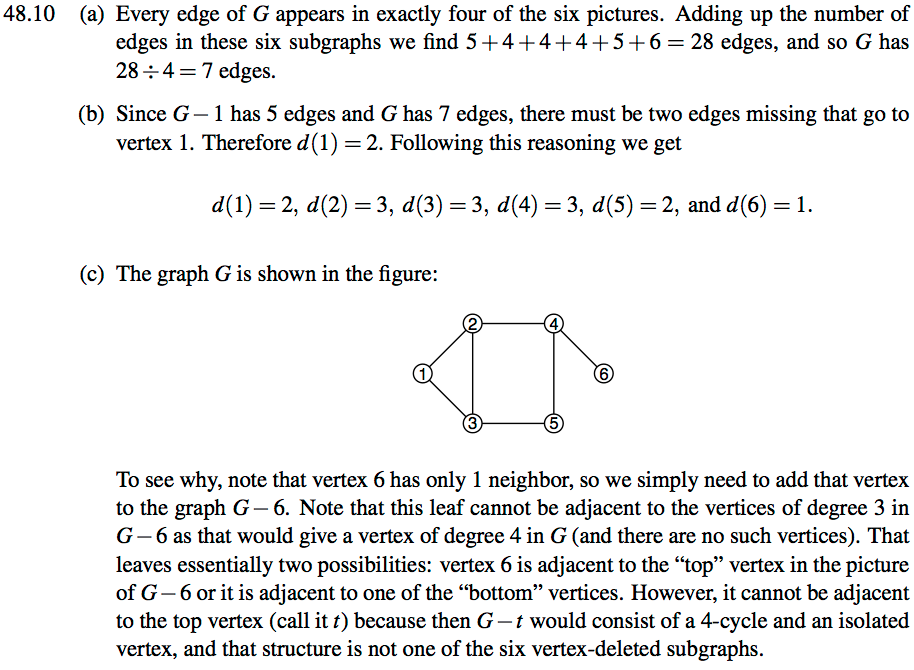•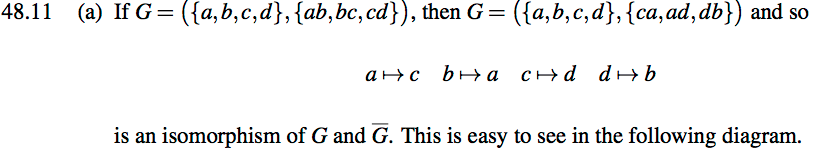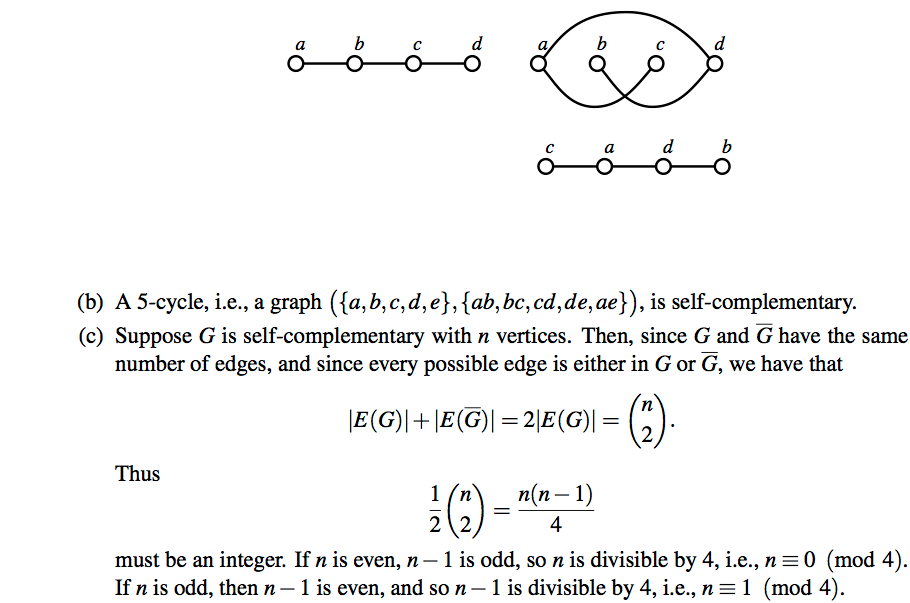;